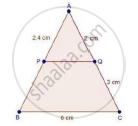Share

# In a δAbc, P and Q Are Points on Sides Ab and Ac Respectively, Such that Pq || Bc. If Ap = 2.4 Cm, Aq = 2 Cm, Qc = 3 Cm and Bc = 6 Cm, Find Ab and Pq. - CBSE Class 10 - Mathematics

ConceptBasic Proportionality Theorem Or Thales Theorem

#### Question

In a ΔABC, P and Q are points on sides AB and AC respectively, such that PQ || BC. If AP = 2.4 cm, AQ = 2 cm, QC = 3 cm and BC = 6 cm, find AB and PQ.

#### SolutionWe have || BC

Therefore, by BPT

We have,

"AP"/"PB"="AQ"/"QC"

2.4/"PB"=2/3

rArr"PB"=(3xx2.4)/2=(3xx24)/2=(3xx6)/5=18/5

⇒ PB = 3.6 cm

Now, AB = AP + PB

= 2.4 + 3.6 = 6cm

Now, In ΔAPQ and ΔABC

∠A = ∠A [common]

∠APQ = ∠ABC [∵ PQ || BC ⇒ Corresponding angles are equal]

⇒ ΔAPQ ~ ΔABC [By AA criteria]

rArr"AB"/"AP"="BC"/"PQ"        [corresponding sides of similar triangles are proportional]

rArr"PQ"=(6xx2.4)/6

⇒ PQ = 2.4 cm

Hence, AB = 6 cm and PO = 2.4 cm

Is there an error in this question or solution?

#### Video TutorialsVIEW ALL 

Solution In a δAbc, P and Q Are Points on Sides Ab and Ac Respectively, Such that Pq || Bc. If Ap = 2.4 Cm, Aq = 2 Cm, Qc = 3 Cm and Bc = 6 Cm, Find Ab and Pq. Concept: Basic Proportionality Theorem Or Thales Theorem.
S